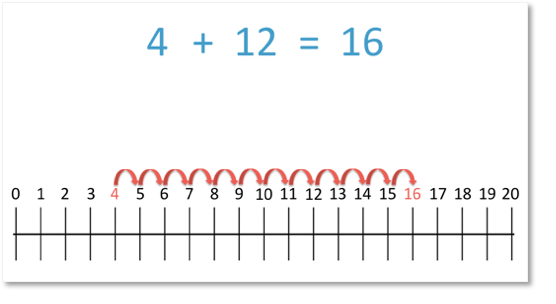# Greater Than and Less Than Signs

Greater Than and Less Than Signs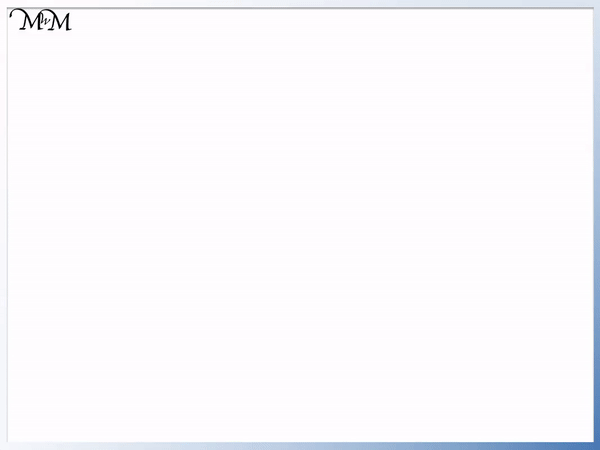• 3 is greater than 1.
• The symbol for ‘greater than’ is ‘>‘.
• We write ‘3 > 1’, which means ‘3 is greater than 1’.
• 6 is less than 8.
• The symbol for ‘less than’ is ‘<‘.
• We write ‘6 < 8', which means '6 is less than 8'.
• We can remember that each comparison symbol points to the smaller number.
• The open end is open to the larger number and we can remember that the crocodile wants to eat the bigger number.
‘>’ means ‘greater than’ and ‘<' means 'less than'.

The open end is facing the bigger number and the arrow points to the smaller number.• 3 is less than 8.
• We can write ‘3 < 8', so that the arrow is pointing at the smaller number.
• The open end of the comparison symbol is facing the 8 because the crocodile wants to eat the larger number.
• 7 is greater than 2.
• We can write ‘7 > 2’, so that the arrow is pointing at the smaller number.
• The open end of the comparison symbol is facing the 7 because the crocodile wants to eat the larger number.# How to Compare Numbers using Greater Than and Less Than Comparison Symbols

In this lesson we are comparing numbers and writing comparison symbols in between the numbers to show which is the larger number.

The symbol for ‘greater than’ is ‘>’.

The symbol for ‘less than’ is ‘<'.

The way to remember which sign to use is that the symbol will point at the smaller number like an arrow.

Another way to remember greater than or less than signs is that the open end of the symbol will be facing the larger number. We can imagine the symbol like a crocodile’s open mouth and then remember that the crocodile will want to eat the largest number to have a larger meal.

In this lesson we will write the comparison symbols of ‘greater than’ and ‘less than’ to compare two numbers.

We will begin with our first comparison symbol, the equals sign.

• Equals Sign (=):

The equals sign means ‘the same value as’.

For example:

3 + 1 = 4

4 = 4

This is telling us that 3 + 1 has the same value as four.• Greater Than Sign (>):

The greater than sign means ‘bigger than’ or ‘larger than’.

For example:

5 > 4

We read this as ‘five is greater than four’.• Less Than Sign (<):

The less than sign means ‘smaller than’.

For example:

4 < 5

This is read as ‘four is less than five’.We will use some examples of greater than or less than signs to look at ways to remember the direction in which the greater and less than signs are drawn when comparing two numbers.

To determine if a number is greater than or less than another number we can look at a number line.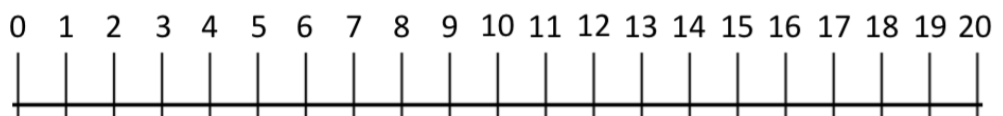The further we go to the right on our number line, the larger our number.

A number is greater than another number if it is further to the right of it on the number line.

Greater Than Example:

In the example below, we can see that 3 is a larger number than 1. So, we say that three is greater than one.

One way that we can remember which sign to put in between the numbers is to think of the comparison sign as an arrowhead. This arrowhead points to the smaller number.

So, it points to 1.Another way to remember which symbol is the greater than sign, is to think of the symbol as a crocodile.

The crocodile is hungry and wants to eat the larger number. So, its mouth opens towards 3.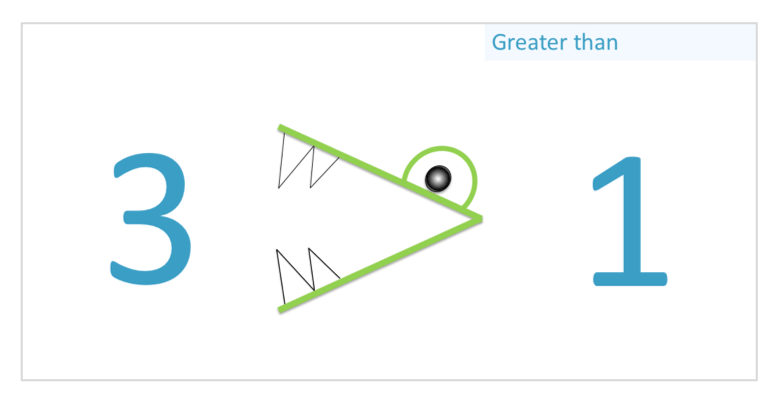Less Than Example:

In the example below, we can see that 6 is a smaller number than 8.

We know this because 6 is on the left of 8 on the number line.

So, we say that six is less than eight.

We can use the same two methods that we used previously to remember which comparison sign represents ‘less than’.

Think of the symbol as an arrowhead which always points towards the smaller number. So, it points towards 6 rather than 8.We could also think of the less than sign as a crocodile that wants to eat the larger number. So, its mouth opens towards 8.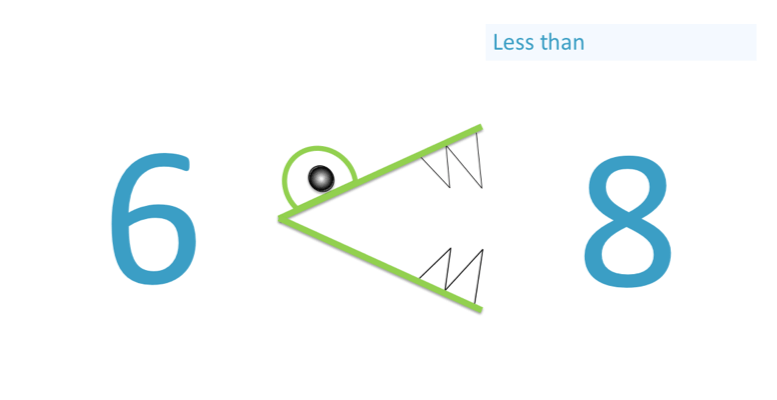When teaching greater than and less than signs, the biggest mistake is writing the symbols the wrong way around.

Fortunately, the signs are the same shape, just in reverse directions.

The best way to remember the direction is that the sign will point at the smallest number like an arrow.Now try our lesson on Addition on a Number Line: Starting at the Largest Number where we learn how to add numbers using a number line.